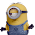## Monday, March 30, 2015

### [Pri20150303VSA] A way to Visualise Arithmetic Progressions

Question
 What is the sum of 25 + 26 + 27 + ......... + 189 ?

Introduction
This is a problem meant to stretch the minds of Singapore primary (elementary) school pupils.  Adults (e.g. parents, teachers and tutors) trying to help out usually recommend doing this using the “rainbow” method (where a bunch of arcs are drawn joining 25 and 189, 26 and 188, 27 and 187 ... and so on, forming something that looks like rainbow), or the sum of arithmetic progression formula
½ ´ number of terms ´ (first term + last term)
which is usually taught at the junior college (pre-university) level.  Although correct, do the learners understand the logic behind them?

A Visual Method
In my visual representation below, the answer pops out almost immediately and the reasoning is made apparent to the student.

Imagine a series of vertical bars representing 25, 26, 27 ... up to 189 joined together forming a staircase (shown in orange).  Make a copy of this (shown in blue) and flip it around and join the two shapes together to form a rectangle.  Note that the width of this rectangle represents the number of terms 189 – 25 + 1 = 165, while the uniform height is in fact first term + last term = 189 + 25 = 214.  Taking the “area” of the rectangle and dividing by two, we get  17 655, an answer that would agree with those found using the previously mentioned methods.  The diagram above is a kind of ”.

1.2.